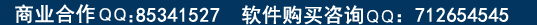正则表达式的语法规则和标记

现在我们正式进入则表达式的学习，我会根据实例结合讲解正则表达式的用法，看完后你就会觉得写ＵＢＢ代码如此简单了，只要你一步一步的跟着我学 看完本文章后你就成为ＵＢＢ高手了。激动人心的就是你能写出自已的ＵＢＢ标签来了，再也不用到别人那里去拷贝现成的代码和模板了。 还好VBScritp5.0给我们提供了“正则表达式”对象，只要你的服务器安装了IE5.x，就可以运行了.

字符描述：

^符号匹配字符串的开头。例如：
^abc　与“abc xyz”匹配，而不与“xyz abc”匹配

\$符号匹配字符串的结尾。例如：
abc\$　与“xyz abc”匹配，而不与“abc xyz”匹配。
注意：如果同时使用^符号和\$符号，将进行精确匹配。例如：
^abc\$　只与“abc”匹配

*符号匹配０个或多个前面的字符。例如：
ab*　可以匹配“ab”、“abb”、“abbb”等

+符号匹配至少一个前面的字符。例如：
ab+　可以匹配“abb”、“abbb”等，但不匹配“ab”。

?符号匹配０个或１个前面的字符。例如：
ab?c?　可以且只能匹配“abc”、“abbc”、“abcc”和“abbcc”

.符号匹配除换行符以外的任何字符。例如：
(.)+　匹配除换行符以外的所有字符串

x|y匹配“x”或“y”。例如：
abc|xyz　可匹配 “abc”或 “xyz”，而“ab(c|x)yz”匹配 “abcyz”和“abxyz”

{n}匹配恰好n次（n为非负整数）前面的字符。例如：
a{2}　可以匹配“aa“，但不匹配“a”

{n,}匹配至少n次（n为非负整数）前面的字符。例如：
a{3,}　匹配“aaa”、“aaaa”等，但不匹配“a”和“aa”。
注意：a{1,}等价于a+
a{0,}等价于a*

{m,n}匹配至少m个，至多n个前面的字符。例如：
a{1,3}　只匹配“a”、“aa”和“aaa”。
注意：a{0,1}等价于a?

[xyz]表示一个字符集，匹配括号中字符的其中之一。例如：
[abc]　匹配“a”、“b”和“c”

[^xyz]表示一个否定的字符集。匹配不在此括号中的任何字符。例如：
[^abc]　可以匹配除“a”、“b”和“c”之外的任何字符

[a-z]表示某个范围内的字符，匹配指定区间内的任何字符。例如：
[a-z]　匹配从“a”到“z”之间的任何一个小写字母字符

[^m-n]表示某个范围之外的字符，匹配不在指定范围内的字符。例如：
[m-n]　匹配除从“m”到“n”之间的任何字符

\符号是转义操作符。例如：
\n　换行符
\f　分页符
\r　回车
\t　制表符
\v　垂直制表符

\\　匹配“\”
\/　匹配“/”

\s　任何白字符，包括空格、制表符、分页符等。等价于“[ \f\n\r\t\v]”
\S　任何非空白的字符。等价于“^\f\n\r\t\v]”
\w　任何单词字符，包括字母和下划线。等价于“[A-Za-z0-9_]”
\W　任何非单词字符。等价于“[^A-Za-z0-9_]”

\b匹配单词的结尾。例如：
ve\b　匹配单词“love”等，但不匹配“very”、“even”等

\B匹配单词的开头。例如：
ve\B　匹配单词“very”等，但不匹配“love”等

\d匹配一个数字字符，等价于[0-9]。例如：
abc\dxyz　匹配“abc2xyz”、“abc4xyz”等，但不匹配“abcaxyz”、“abc-xyz”等

\D匹配一个非数字字符，等价于[^0-9]。例如：
abc\Dxyz　匹配“abcaxyz”、“abc-xyz”等，但不匹配“abc2xyz”、“abc4xyz”等

\NUM匹配NUM个（其中NUM为一个正整数），引用回到记住的匹配。例如：
(.)\1　匹配两个连续相同的字符。

\oNUM匹配n（其中n为一个小于２５６的八进制换码值）。例如：
\o011　匹配制表符

\xNUM匹配NUM（其中NUM为一个小于２５６的十六进制换码值）。例如：
\x41　匹配字符“A”

1）在字符串中精确查找链接地址

((http|https|ftp):(\/\/|\\\\)((\w)+[.]){1,}(net|com|cn|org|cc|tv|[0-9]{1,3})(((\/[\~]*|\\[\~]*)
(\w)+)|[.](\w)+)*(((([?](\w)+){1}[=]*))*((\w)+){1}([\&](\w)+[\=](\w)+)*)*)

以http://或者https://或者ftp://等开头（当然还有其它形式，这里只列出主要的）

http://后面必须跟一个单词字符，紧接着单词字符后面的是"."（这样的组合必须出现一次或多次）。紧跟着“.”后面的是域名后缀（如net或者com或者cn等，如果是以IP地址的形式出现就可以是数字）

出现完整的链接地址后，还可以出现下一级或者更多级的目录（还要注意个人主页的地址有可能出现"~"符号）

链接地址末尾可以带参数。如典型的页数?PageNo=2&action=display等

1、((http|https|ftp):(\/\/|\\\\) 满足条件１

2、((\w)+[.]){1,}(net|com|cn|org|cc|tv|[0-9]{1,3}) 满足条件２
“((\w)+[.]){1,}”表示一个单词字符加一个点号可以出现1次或者多次（这里考虑了某些用户喜欢省略www而将http://www.w3c.com写成http://w3c.com）
“(net|com|cn|org|cc|tv|[0-9]{1,3})”表示必须要以net或者com或者cn或者org或者cc或者tv或者三位以下的数字结束
[0-9]{1,3}表示三位以下的数字，因为ip地址的任何段不能超过255

3、(((\/[\~]*|\\[\~]*)(\w)+)|[.](\w)+)* 满足条件３
“(\/[\~]*|\\[\~]*)”表示可以出现"/~"或者是"\~"，（其中“[\~]*”表示 ~ 可以出现也可以不出现），因为不是每个链接地址都有下一级目录
“(\w)+)|[.](\w)+)”表示必须出现一个单词字符（即目录或者是一个带有扩展名的文件）

4、(((([?](\w)+){1}[=]*))*((\w)+){1}([\&](\w)+[\=](\w)+)*)*)满足条件４
“((([?](\w)+){1}[=]*))*((\w)+){1}”表示形如"?PageNo=2"的字符串可以出现也可以不出现，如果出现则只能出现一次（因为不可能有两个“？”号出现）。

“([\&](\w)+[\=](\w)+)*)”表示形如“&action=display”的字符串可以出现也可以不出现（因为并不是每个网页都带有两个以上的参数。

2）替代典型的UBB标签:[/b]

(\[b\])(.+)(\[\/b\])

str=checkexp(re,str,"<b>\$2</b>")
（注意：checkexp是我自定义的函数，将在后面给出。这个函数将把[/b]按照我们提供的模板进行替代。）

Function ReThestr(face,str)
dim re,str

re="\>"
str=checkexp(re,str,"&gt;")

re="\<"
str=checkexp(re,str,"&lt;")

re="\n\r\n/"
str=checkexp(re,str,"<P>")

re=chr(32)
str=checkexp(re,str,"&nbsp;")

re="\r"
str=checkexp(re,str," ")

re="\[img\]((http:(\/\/|\\\\)){1}((\w)+[.]){1,3}(net|com|cn|org|cc|tv)(((\/[\~]*|\\[\~]*)
(\w)+)|[.](\w)+)*(\w)+[.]{1}(gif|jpg|png))\[\/img\]" ‘’‘’查找图片地址
str=checkexp(re,str," <img src=‘’‘’\$1‘’‘’> ")

re="\[w\](http:(\/\/|\\\\)((\w)+[.]){1,}(net|com|cn|org|cc|tv)(((\/[\~]*|\\[\~]*)(\w)+)|[.](\w)+)*
(((([?](\w)+){1}[=]*))*((\w)+){1}([\&](\w)+[\=](\w)+)*)*)\[\/w\]" ‘’‘’查找帧地址
str=checkexp(re,str,"<iframe width=‘’‘’300‘’‘’ height=‘’‘’300‘’‘’ src=‘’‘’\$1‘’‘’></iframe>")

re="([^(‘’‘’>)])(<br>)*((http|https|ftp):(\/\/|\\\\)((\w)+[.]){1,}(net|com|cn|org|cc|tv|([0-9]{1,3}))(((\/[\~]*|\\[\~]*)(\w)+)|[.](\w)+)*(((([?](\w)+){1}[=]*))*((\w)+){1}([\&](\w)+[\=](\w)+)*)*)" ‘’‘’查找链接地址
str=checkexp(re,str,"\$1\$2 <a href=‘’‘’\$3‘’‘’ target=_blank>\$3</a> ")

re="([^(http://|http:\\)])((www|cn)[.](\w)+[.]{1,}(net|com|cn|org|cc)(((\/[\~]*|\\[\~]*)(\w)+)|[.](\w)+)*
(((([?](\w)+){1}[=]*))*((\w)+){1}([\&](\w)+[\=](\w)+)*)*)" ‘’‘’查找不以http://开头的地址
str=checkexp(re,str,"\$1 <a href=‘’‘’http://\$2‘’‘’ target=_blank>\$2</a> ")

re="([^(=)])((\w)+[@]{1}((\w)+[.]){1,3}(\w)+)" ‘’‘’查找邮件地址
str=checkexp(re,str," <a href=‘’‘’mailto:\$2‘’‘’>\$2</a> ")

re="\[0-F]{6})\]((.)+)\[\/color\]" ‘’‘’替换字体色彩
str=checkexp(re,str,"<font color=‘’‘’\$1‘’‘’>\$4</font>")

re="\{1})\]((.)+)\[\/size\]" ‘’‘’替换字体大小
str=checkexp(re,str,"<font size=‘’‘’\$1‘’‘’>\$2</font>")

re="\((.)+)\[\/font\]" ‘’‘’替换字体
str=checkexp(re,str,"<font face=‘’‘’\$1‘’‘’>\$3</font>")

re="(\[b\])(.+)(\[\/b\])" ‘’‘’加粗字体
str=checkexp(re,str,"<b>\$2</b>")

re="(\[u\])(.+)(\[\/u\])" ‘’‘’下画线
str=checkexp(re,str,"<u>\$2</u>")

re="(\[li\])(.+)(\[\/li\])" ‘’‘’列表
str=checkexp(re,str,"<li>\$2</li>")

re="(\[QUOTE\])(.+)(\[\/QUOTE\])" ‘’‘’引用
str=checkexp(re,str,"<BLOCKQUOTE>引用:<HR SIZE=1>\$2<HR SIZE=1></BLOCKQUOTE>")

re="\){1,3}(\w)+)\](.+)(\[\/email\])" ‘’‘’邮件
str=checkexp(re,str,"<a href=mailto:\$1>\$6</a>")

re="(\[center\])(.+)(\[\/center\])" ‘’‘’居中
str=checkexp(re,str,"<center>\$2</center>")

re="fuck"
str=checkexp(re,str,"***")

re="操"
str=checkexp(re,str,"***")

re="sex"
str=checkexp(re,str,"***")

re="TMD"
str=checkexp(re,str,"***")

re="shit"
str=checkexp(re,str,"***")

ReThestr=str
end function

ＵＢＢ代码如下：

[url] [/url] [email=] [img] [/img]

--------------------------------------------------------------------------------

--------------------------------------------------------------------------------

[li] [/li] [font=impact] [color=Yellow] [size=4]

[img] [/img]http://cn.yahoo.com
http://aol.com http://192.168.0.1
www.yahoo.com how are you[/b]
http://page2000.xiloo.com/~page2000?PageNo=2&action=del
lucaihui@cmmail.com 大家好http:\\page2000.shit
<font color=red>http://test.com</font>http://test

Function CheckExp(patrn,strng,tagstr)
Dim regEx,Matches

Set regEx=New RegExp‘’‘’建立一个新对像
regEx.Pattern=patrn‘’‘’设置模板
regEx.IgnoreCase=true‘’‘’搜索是否区分大小写的 true表是不区分 flase表示区分
regEx.Global=True‘’‘’搜索是否应用于整个字符串

Matches=regEx.replace(strng,tagstr)‘’‘’匹配并替代字符串

CheckExp=Matches返回函数结果
end function

text=ReThestr(text)

content=replace(content,vbcrlf,"<br />")

vbCrlf则回车换行符号。